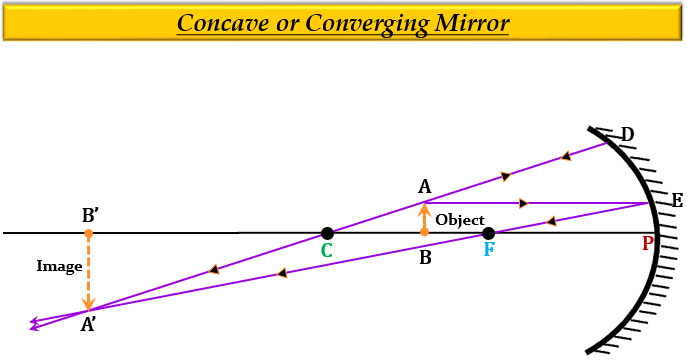# (a) An object is placed just outside the principal focus of concave mirror. Draw a ray diagram to show how the image is formed, and describe its size, position and nature.(b) If the object is moved further away from the mirror, what changes are there in the position and size of the image?(c) An object is 24 cm away from a concave mirror and its image is 16 cm from the mirror. Find the focal length and radius of curvature of the mirror, and the magnification of the image.

(a) Ray Diagram- to show how the image is formed when an object is placed just outside the principal focus of the concave mirror.The size of the image is magnified, the position of the image is beyond the center of curvature $(C)$ of the mirror, and the nature of the image is real and inverted.

(b) If the object is moved further away from the mirror, the image will move towards the mirror and its size will decrease gradually.

(c) Given:

Distance of object, $u$= $-$24 cm

Distance of image, $v$= $-$16 cm

To find: Focal length of the mirror $(f)$, radius of curvature of the mirror $(R)$, and the magnification of the image, $(m)$.

Solution:

From the mirror formula, we know that-

$\frac{1}{f}=\frac{1}{v}+\frac{1}{u}$

Substituting the given values in the mirror formula we get-

$\frac{1}{f}=\frac{1}{(-24)}+\frac{1}{(-16)}$

$\frac{1}{f}=-\frac{1}{24}-\frac{1}{16}$

$\frac{1}{f}=\frac{-2-3}{48}$

$\frac{1}{f}=\frac{-5}{48}$

$f\times {(-5)}=48$

$f=-\frac{48}{5}$

$f=-9.6cm$

Thus, the focal length $f$ of the concave mirror is 9.6 cm.

For radius of curvature $(R)$, we know that-

$R=2f$, where R = Radius of curvature, and f = Focal length

$R=2\times {(-9.6)}$

$R=2\times {(-9.6)}$

$R=-19.2cm$

Thus, the radius of curvature $(R)$ of the mirror is 19.2 cm.

Now, from the magnification formula, we know that-

$m=-\frac{v}{u}$

Substituting the given values in the magnification formula we get-

$m=-\frac{(-16)}{-24}$

$m=\frac{(16)}{-24}$

$m=-0.66$

Thus, the magnification $(m)$, of the mirror is 0.66.

Hence, the image is real, inverted, and small in size.

Updated on: 10-Oct-2022

1K+ Views